When the sides of a rhombus ABCD are 10 cm and 24 cm, respectively, what is the perimeter of the shape?

# When the sides of a rhombus ABCD are 10 cm and 24 cm, respectively, what is the perimeter of the shape?

1. A
52 square units
2. B
54 square units
3. C
50 square units
4. D
56 square units

Fill Out the Form for Expert Academic Guidance!l

+91

Live ClassesBooksTest SeriesSelf Learning

Verify OTP Code (required)

### Solution:

Concept: Drawing the rhombus first will allow us to use the Pythagorean Theorem to determine the side length of the rhombus, which we will then multiply by 4 to obtain the perimeter.
To better comprehend it, let's first illustrate it.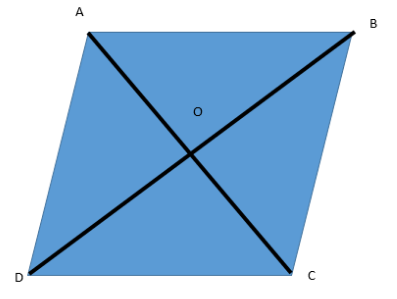Here, BD is 24 cm and AC is 10 cm.
We are aware that rhombus' diagonals cut each other at right angles.
AOB, BOC, COD, and DOA are all right-angled triangles as a result.
The diagonals are split because of O. AO = OC and DO = OB as a result.
These together with the diagonal lengths give us AO = OC = 5 cm and DO = OB = 12 cm.
As stated by the Pythagorean Theorem, "In a right-angled triangle, the square of the hypotenuse side is equal to the sum of the squares of the other two sides."
Because AOB is a triangle with a right angle at O, it has a right angle. The Pythagorean Theorem will result in the following: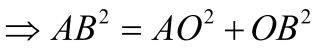Putting in the values, we get:-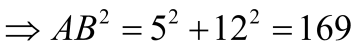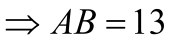The side of the rhombus is 13 units as a result.
Since a rhombus's perimeter is determined by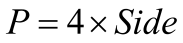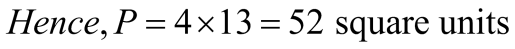Hence, the correct option is 1) 52 square units

## Related content

 Area of Square Area of Isosceles Triangle Pythagoras Theorem Triangle Formula Perimeter of Triangle Formula Area Formulae Volume of Cone Formula Matrices and Determinants_mathematics Critical Points Solved Examples Type of relations_mathematics+91

Live ClassesBooksTest SeriesSelf Learning

Verify OTP Code (required)